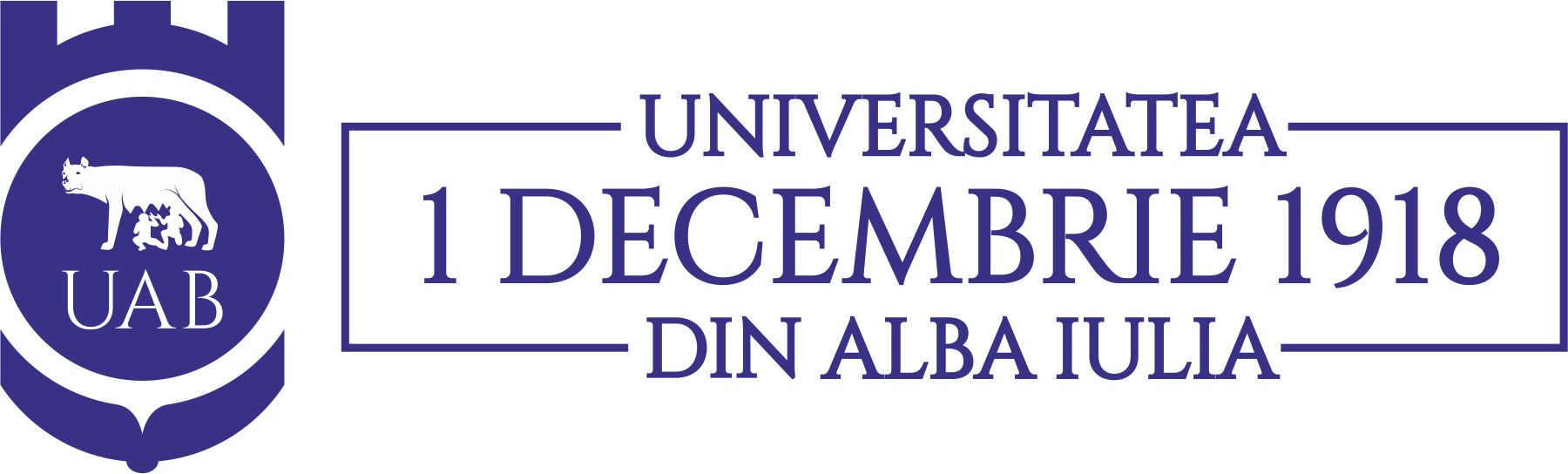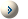Acta Universitatis Apulensis

Universitatea „1 Decembrie 1918” din Alba Iulia

Year 2014 / Acta / 39

SUMMARYA. Ayoujil -- On class of nonhomogeneous discrete Dirichlet problemsA. Latifi, A. Shakoori Samad -- New proofs for theorems proven by Shiraishi and OwaA. Alb Lupas -- On univalence criteria for analytic functions defined by Salagean operator and Ruscheweyh derivativeZ. Ayati, J. Biazar, B. Gharedaghi -- The application of modified homotopy analysis method for solving linear and non-Linear inhomogeneous Klein-Gordon equationsL.K. Dey, S. Mondal, S.N. Mishra -- Cantor"s intersection theorem and Baire"s category theorem in generalized metric spaceS. Kumar, S.S. Bhatia, V. Kumar -- Lacunary strongly almost summable sequence spaces defined by ideal and modulus functionN. Marikkannan -- A subclass of analytic functions and a generalized linear differential operatorM. Gulistan, M. Shahzad, N. Yaqoob -- On  (e; e VQK )-Fuzzy KU-ideals of KU-algebrasO.R. Sayed -- On preserving OmegaS-closeness in topological spacesP. Sharma, S. Porwal, A. Kanaujia -- A generalized class of Harmonic Univalent Functions associated with Salagean Operators Involving ConvolutionsM.A. Pathan, Waseem A. Khan -- Some implicit summation formulas and symmetric identities for the generalized Hermite-based polynomialsQ. Din -- On a system of fourth-order rational difference equationsR. Kumar, G. Prema, B. S. Kiranagi -- Lie algebra bundles of finite typeShyamal Kumar Hui -- On phi-pseudo symmetric Para-Sasakian manifoldsU. Chand De, S. Ghosh -- A classification of three dimensional N(k) contact metric manifoldsC. Selvaraj, T.R.K. Kumar, G. Thirupathi -- Coefficient bounds for a subclass of Bi-univalent functionsT.M. Seoudy -- Some subordination theorems for certain subclasses of analytic functions involving a linear operatorC. Selvaraj, G. Thirupathi -- Coefficient bounds for a subclass of Bi-univalent functions using Salagean operatorK.R. Vasuki, R.G. Veeresha, Khaled A.A. Alloush -- Further two dimensional series evaluations via the elliptic functions of Ramanujan and JacobiA.O. Mostafa, M.K. Aouf, A. Shamandy and E.A. Adwan -- Subclass of Harmonic Univalent Functions Defined by Modified Cata"s OperatorC. Patra and A. Bhattacharyya -- Deformation of an LSP-Sasakian ManifoldA. Neirameh -- Complex wave behavior to the TDB and (2 +1)-DZ equationsN. Ahmad, A. Zada, I.U. Khan -- Discrete Characterization of Exponential Stability of Evolution Family Over Hilbert SpaceD. Breaz, V. Pescar -- On conditions for univalence of some integral operatorsD.O. Ajayi, T.C. Adefokun -- Sufficient Conditions for Hamiltoniancity of Certain Special GraphsG.S. Saluja -- Convergence theorems for generalized asymptotically quasi-nonexpansive mappings in CAT(0) spacesH. Shayanpour -- *-Ring n-homomorphisms between topological algebrasI.-L. Popa, M. Megan, T. Ceausu -- On h-Trichotomy of Linear Discrete-Time Systems in Banach SpacesS. Modak, B. Garai -- Note on continuities in minimal spaces